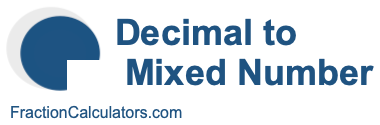What is 2.501 as a mixed number?Here we will show you how to convert the decimal number 2.501 to a mixed number. In other words, we will show you how to convert 2.501 to a whole number (W) and a fraction with a numerator (N) and a denominator (D). Here is the illustration of what we are going to convert:

2.501 ->> Mixed Number
2.501 ->> W N/D

Before we continue, and in case you are wondering, note that mixed number is the same as mixed fraction. Thus, 2.501 as a mixed number is the same as 2.501 as a mixed fraction. Anyway, here are step-by-step instructions showing you how to get 2.501 as a mixed number:

The whole number is the number to the left of the decimal point. Therefore, the whole number (W) is equal to 2.

The numerator is the number to the right of the decimal point. Therefore, the numerator (N) is 501.

The get the denominator, you take 10 to the power of the number of digits in the numerator. There are three digits in the numerator and 10^3 is 1000, which means that the denominator is 1000.

When we put it all together and simplify if necessary, we get the answer to 2.501 as a mixed number, like this:

2.501 = 2 501/1000
2.501 = 2 501/1000
2.501 as a mixed number = 2 501/1000

Decimal to Mixed Number Calculator
Use this tool to convert another decimal number to a mixed number.

What is  as a mixed number?

What is 2.502 as a mixed number?
Here is another decimal number that we have converted to a mixed number for you.